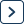Linear Algebra: Matrices

# Linear Algebra: Matrices - Mathematical Models | Physics | Notes, Videos & Tests

Linear Algebra: Matrices is topic-wise collection of Important notes, Topic Wise tests, Video lectures, NCERT Textbook, NCERT Solution, and Previous Year papers is designed in a way where you get a complete chapter-wise package for your preparation of Mathematical Models in one place? Here, the chapter-wise guide is framed by the best teachers having tremendous knowledge in the respective streams, thereby making the Linear Algebra: Matrices - Mathematical Models the ultimate study source for the chapter.
Linear Algebra: Matrices - Mathematical Models | Physics | Notes, Videos & Tests is part of Physics 2023 for Mathematical Models preparation. The notes and questions for Linear Algebra: Matrices - Mathematical Models | Physics | Notes, Videos & Tests have been prepared according to the Physics exam syllabus. Information about Linear Algebra: Matrices - Mathematical Models | Physics | Notes, Videos & Tests covers all important topics for Physics 2023 Exam. Find important definitions, questions, notes, meanings, examples, exercises and tests below for Linear Algebra: Matrices - Mathematical Models | Physics | Notes, Videos & Tests. Introduction of Linear Algebra: Matrices - Mathematical Models | Physics | Notes, Videos & Tests in English is available as part of our Mathematical Models for Physics & Linear Algebra: Matrices - Mathematical Models | Physics | Notes, Videos & Tests in Hindi for Mathematical Models course. Download more important topics related with Linear Algebra: Matrices - Mathematical Models | Physics | Notes, Videos & Tests, notes, lectures and mock test series for Physics Exam by signing up for free.
In this chapter you can find the Linear Algebra: Matrices - Mathematical Models | Physics | Notes, Videos & Tests defined & explained in the simplest way possible. Besides explaining types of Linear Algebra: Matrices - Mathematical Models | Physics | Notes, Videos & Tests theory, EduRev gives you an ample number of questions to practice Linear Algebra: Matrices - Mathematical Models | Physics | Notes, Videos & Tests tests, examples and also practice Physics tests.
 1 Crore+ students have signed up on EduRev. Have you?

## Notes for Linear Algebra: Matrices - Mathematical Models | Physics

Linear Algebra: Matrices Notes for Physics is part of Mathematical Models Notes for Quick Revision. These Linear Algebra: Matrices sections for Mathematical Models Notes are comprehensive and detailed yet concise enough to glance through for exam preparations. The Linear Algebra: Matrices Topic is one of the critical chapters for Physics aspirants to understand thoroughly to perform well in the Mathematical Models Section of the Physics Examination. Many aspirants find this section a little complicated and thus they can take help from EduRev notes for Physics, prepared by experts according to the latest Physics syllabus.

## Notes for Linear Algebra: Matrices - Mathematical Models | Physics

After completing the Linear Algebra: Matrices it becomes important for students to evaluate themselves how much they have learned from the chapter. Here comes the role of the chapter-wise Test of Linear Algebra: Matrices. EduRev provides you with three to four tests for each chapter. These MCQs (Multiple Choice Questions) for Physics are designed to make them understand the types of questions that come during the exam. By attempting these tests one can not only evaluate themselves but can also make a good hold on Mathematical Models. Taking tests helps them manage time during the exam and also builds their confidence. For proper learning, we have provided here a number of Tests. Taking these tests will definitely help them improve your score.

## MCQ of Linear Algebra: Matrices

MCQs are the questions in which students of Physics can gain marks or can lose marks very easily. These questions are One marks questions with given multiple choices. They need to choose the correct option. These questions are very simple just based on the basic concepts. All they need to do is to make their concept very clear by learning the study material available for Mathematical Models Linear Algebra: Matrices at EduRev. Learning these MCQ (Multiple choice Questions) questions will definitely help them improve their score in the exam. Some MCQ type questions are provided here for practise:

## Other Chapters in Mathematical Models for Physics

The Complete Chapterwise preparation package of Mathematical Models is created by the best Physics teachers for Physics preparation. 22202 students are using this for Physics preparation.
Linear Algebra: Matrices | Mathematical Models
 01 Vector Analysis5 Docs | 5 Tests02 Ordinary Differential Equation3 Docs | 4 Tests03 Fourier Series3 Docs | 2 Tests04 Calculus of Single & Multiple Variables2 Docs | 4 Tests05 Complex Numbers2 Docs | 5 TestsPart of the course
 Mathematical Models 18 docs | 24 tests(Scan QR code)